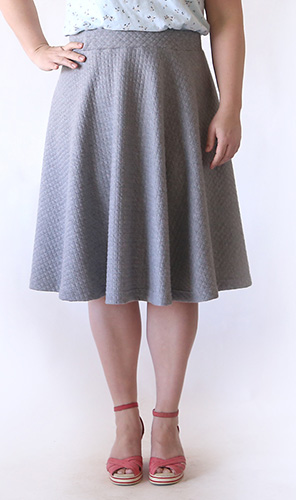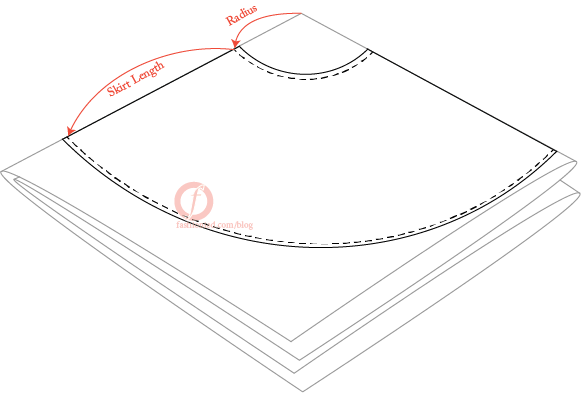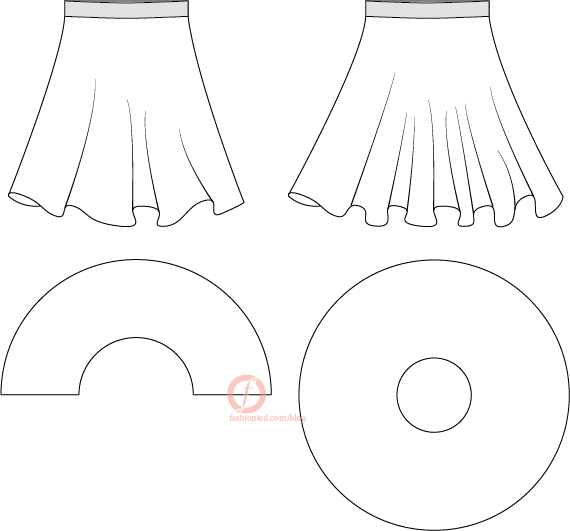# Circle Skirt Pattern TutorialDrawing the circle skirt pattern is very easy, yet it provide a lot of options that allow many variations, also it is suitable for any beginner and fast to do too. The drawing principles can be modified in order to draw:

• Pleated circle skirt. Yes, it is possible.
• Circle skirt with fullness.
• Plus size circle skirt pattern.
• Circle skirt with deferent lengths between front/side/back.

In addition to many more ideas, actually nothing limit your circle skirt creations but your own creativity boundaries.

Basic circle skirt is nothing but a circle or part of a circle, the basic idea is to draw a circle with Circumference equals (usually) to the waist measurement. In order to do this, it is important to have in mind the relation between circle radios and circumference. As most of us know there is a mathematical relation between them as follow:

• The Radius is the distance from the center outwards.
• The circumference is the distance once around the circle.
• The relation is known; circumference = 2 π r

## The basic full circle skirt pattern

Let us assume we are drawing a circle skirt with the measurements:

• Waist= 30”
• Length= 40”

Since we know the circumference, while π is known constant equals 3.14, we only need to find the radios (r), as follow:

r = circumference / 2 π = circumference / 6.28

I like to keep things simple and just divide circumference by 6. For the above skirt measurements, the waist is 30” when it is divided by 6 the result = 5” so if we draw a circle with that radios the circumference should be almost 30” that is it, so simple, right?

In fact, I have few points here to mention:

• First: the circumference will be a bit longer than 30”, which is ok, the extra length will be squeezed on machine while sewing, this extra length is needed and must be included in every skirt what so ever.
• Second: here we are drawing the pattern without and seam allowance, make sure to add seam allowance to each line (going to be sewn) in the pattern, seam allowance is usually 0.5” however, you are free to add any amount you prefer as long as you are going to sew exactly this amount later. Circle skirt’s hem usually are not double folded, rather it’s overlocked and folded once, hence it’s usual to add only 1/4″ for the fold.
• Third: so far, we just drew the waistline, we need to draw hem line to finish the skirt.

To draw hem line, sum the radios length 5” we calculated earlier and the skirt length 40’, 40 + 5 = 45”, then we draw another circle from the same center used to draw the first one. The area between the two circles is the actual pattern.Finally add seam allowance to waistline and hem  line, we usually add les seam allowance at circle skirt pattern hem  line, because it is hard to make double fold here, the easiest finish is to over-lock the edge then fold it once on single needle machine.

## Half circle skirt

Full circle hem line is very wide, looks very nice on body and require much fabric too, sometimes a simpler version is needed, here we can modify the steps a little to make a simplified edition.Using exactly same measurements used for full circle skirt, we just double the waist measurement, and then we follow on same steps, however we cut half the circle only not a full circle. Since we doubled the waist measurement, half the circle circumference will be equal to real waist measurement, hence half a circle will make one skirt, but not like full circle skirt, and this one will provide less fullness.

## Circle skirt with fullness

Imagine if you use the above pattern (half circle skirt pattern) and cut the full circle. The skirt’s waist is going to be as double as real waist measurement, right? What if we gather all this fabric and make fullness? Simple.

In case the required fullness is not exactly double the fabric, we are going to increase the original waist measurement by percentage, for example, a skirt for 30” waist may be drawn as 45” (30 * 1.5) in order to add 15” of extra fabric and so on.

## Plus size circle skirt

What is cool about circle skirts is that you do not need to do so much math to make it fit for plus sized bodies, just measure the waist as it is and do the simple math.

## Circle skirt with different lengths between front/side/back.

I like this one; it is where the real joy begins, here you can make short skirts at front and longer at back, or may be shorter at one side seam, may be both !!

Whatever is the required shape, the idea is basic, first we have to draw the circle skirt in full shape if it’s asymmetric pattern, or to draw it half shape if it is not.

Then, we do not draw both circles (waistline – hemline) on the same center, we shift one of them (the hemline circle) towards the longer length. Therefore, if we want to draw a shorter front circle skirt, we shift hemline circle half the deference between the front and back lengths.

Suppose we are drawing a 30” skirt, simple draw a 5” (30/6) radius circle, and if the skirt is 30” front length and 50” back length, then we will set the length average as 40” (30+50/2), but when drawing that hemline skirt, we will shift its center 10” (50-30/2) towards back.

## summary

In this post I try to show the basic idea behind the circle skirt pattern making, in addition to few ideas and variations that can be developed from this basic idea, actually the ideas for circle skirts are not limited and they are too many to show them in single post. I try here to show the most common ideas only, however, if you believe that I forget to show one of them please let me know, I will add it to this post. In addition, if you have any question just leave a comment, I will reply it ASAP.